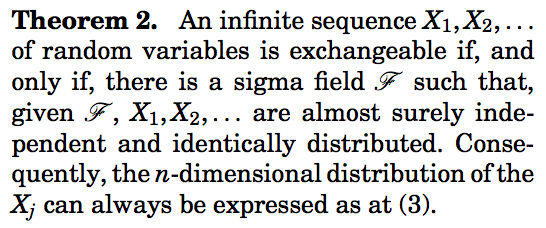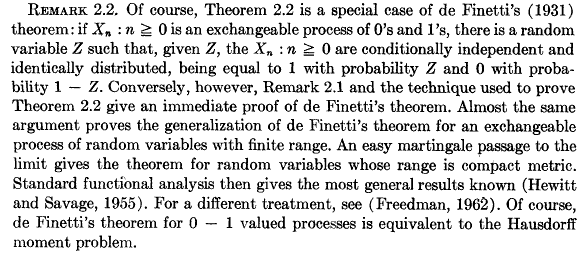# Correlations, dimension, and risk measure

Yesterday, while I was attending the IFM2 conference, at HEC Montreal, I heard a nice talk about credit risk, and a comparison between contagion (or at least default correlation), for corporate and retail companies (in the US). And it was mentioned that default correlation was much lower for retail companies than it could be for corporate risk. In a discussion that followed those slides, it was mentioned that banks in the US should actually have been working more with those small firms, since contagion risk was much lower.

A problem here is that the link between correlation, risk and dimension is rather complicated:

• corporate means a small number of firms, high correlation (and possible large individual losses)
• retail means a large number of firms (even perhaps extremely large), lower correlation (and small individual losses)

A simple model for default models is based on the assumption that we deal with an exchangeable portfolio (as in a previous post). With the following code, given an (individual) default probability, a default correlation, and a number of firms, it is possible to calculate the probability to have more than a given number of defaults.

``` proba=function(s,a,m,n){
b=a/m-a
choose(n,s)*integrate(function(t){t^s*(1-t)^(n-s)*
dbeta(t,a,b)},lower=0,upper=1,subdivisions=1000,
stop.on.error =  FALSE)\$value}

CDF=function(x=10,r=.4,m=.1,n=50){
a=m*(1-r)/r ;
V=rep(NA,n+1)
for(i in 0:n){
V[i+1]=proba(i,a,m,n)}
V=V/sum(V);
return(sum(V[1:(x+1)])) }```

It is possible to calculate, for a large range of correlations, the probability to have – at least – 20% of default in the portfolio (in order to compare things that are comparable).

```R=seq(.01,.99,by=.01)
VQ=matrix(NA,length(A),2)
for(i in 1:length(A)){
VQ[i,1]=1-CDF(r=A[i],x=4,n=20);
VQ[i,2]=1-CDF(r=A[i],x=200,n=1000)}```

With 20 firms (corporate) we want to have at least 4 defaults, while with 1000 firms (retail) there should be 200 defaults. As mentioned in the previous post, the relationship between correlation and quantiles of sums is not simple. Hence, it might not be monotone. The dotted line is the probability to have at least 4 defaults when default correlation is 50% (around 10%). The plain line is the probability to have at least 200 defaults, as a function of the correlation,

```plot(A,1-VQ[,2],type="l",col="red",ylim=c(0,.22))
abline(h=1-VQ[50,1],lty=2,col="red")```In that case, with only a correlation of 10% among retail firms, the probability of having 20% defaults is the same as the same probability for corporate, but with 50% correlation… One should remember that in portfolio analysis, the links between correlation, dimension and risk measure is a sensitive issue…

# de Finetti’s theorem and exchangeability

This week, we will start to work on multivariate models, and non-independence. The first idea to discuss non-independence will be to use the concept ofexchangeability. A sequence of random variableis said to be exchangeable if for allfor any permutationof. A standard example is the case where, withandSince, a necessary condition is thati.e.Since this inequality should hold for allit comes that necessarily.
de Finetti (1931): Letbe a sequence of random variables with values inis exchangeable if and only if there exists a distribution functiononsuch thatwhere. Note thatis the distribution function of random variableA nice proof of that result can be found in Heath & Sudderth (1995) – see alsoSchervish (1995)Chow & Teicher (1997) or Durrett (2010) and also probably in several bayesian books because that result has a strong interpretation in bayesian inference (as far as I understood, see e.g. Jaynes (1982)).
From the exchangeability condition, for any permutationof,that can be inverted inThe idea is then to extend the size of the vector, i.e. for all, defineso that, if we condition on,but since given the sum of components of, all possible rearrangements of the ones among theelements are equally likely, we can writeThe first idea is to work on the blue term, and to invocate a theorem of approximation of the hypergeometric distributionto a binomial distribution, whenbecomes large. ThenLetand letdenote the cumulative distribution function of.The idea is then to write the sum as an integral, with respect to that distribution,The theorem is then obtained since, i.e.In the case of non-binary sequences, there is an extension of the previous result,
Hewitt & Savage (1955): Letbe a sequence of random variables with values in.is exchangeable if and only if there exists a measureonsuch thatwhereis the measure associated to the empirical measureandFor instance, in the Gaussian case mentioned earlier, ifthenwherei.e. conditionally on, theare conditionally independent, with distribution. The proof can be found in Kingman (1978) and is based on martingale arguments.
Note that in the Gaussian case,whereare i.i.d. random variables. To go further on exchangeability and related topics, see Aldous (1985)  (see also here).
This construction can be used in credit risk, to model defaults in an homogeneous portfolio, see e.g. Frey (2001),Assuming a Beta distribution for the latent factor, we can derive the probability distribution of the sumSinceif we assume that – given the latent factor –(either the company defaults, or not),i.e.Thus, we can derive the (unconditional) distribution of the sumi.e.```> proba=function(s,a,m,n){
+ b=a/m-a
+ choose(n,s)*integrate(function(t){t^s*(1-t)^(n-s)*
+ dbeta(t,a,b)},lower=0,upper=1)\$value
+ }```

Based on that function, it is possible to plot the probability distribution over. In the upper corner is plotted the density of the Beta distribution.

```> a=2
> m=.2
+ n=10
+ V=rep(NA,n+1)
+ for(i in 0:n){
+ V[i+1]=proba(i,a,m,n)}
> barplot(V,names.arg=0:10)```Those two theorems are extremely close,

De Finetti’s theorem: a random sequenceofrandom variables is exchangeable if and only if‘s are conditionnally independent, conditionnally on some random variable.

Hewitt-Savage’s theorem: a random sequenceis exchangeable if and only if‘s are conditionnally independent, conditionnally on some sigma-algebraOlshen (1974), proposed an interesting discussion about those theorems, see also in the Encyclopedia of Statistical Science,The subtle difference between those two theorem is also discussed in Freedman (1965)